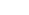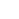Measures of Dispersion in Statistics; Types and Examples

# Measures of Dispersion in Statistics; Types and Examples

Published
15th Sep, 2023
Views7 Mins## What is Dispersion in Statistics

Dispersion in statistics is a way of describing how to spread out a set of data is. Dispersion is the state of data getting dispersed, stretched, or spread out in different categories. It involves finding the size of distribution values that are expected from the set of data for the specific variable. The meaning of dispersion in statistics is “numeric data that is likely to vary at any instance of average value assumption”.

Dispersion of data in Statistics helps one to easily understand the dataset by classifying them into their own specific dispersion criteria like variance, standard deviation and ranging.

Dispersion is a set of measures that helps one to determine the quality of data in an objectively quantifiable manner. Most often data science courses start with the basics of statistics and dispersion is one such concept that you cannot afford to skip.

## Measures of Dispersion

The measures of dispersion contain almost the same unit as the quantity being measured. There are many Measures of Dispersion found that help us to get more insights into the data:

1. Range
2. Variance
3. Standard Deviation
4. Skewness
5. IQRImage Source

### Types of Measures of Dispersion

The Measure of Dispersion in Statistics is divided into two main categories and offer ways of measuring the diverse nature of data. It is mainly used in biological statistics. We can easily classify them by checking whether they contain units or not.

So as per the above, we can divide the data into two categories which are:

• Absolute Measures of Dispersion
• Relative Measures of Dispersion## Absolute Measures of Dispersion

Absolute Measures of Dispersion is one with units; it has the same unit as the initial dataset. Absolute Measure of Dispersion is expressed in terms of the average of the dispersion quantities like Standard or Mean deviation. The Absolute Measure of Dispersion can be expressed in units such as Rupees, Centimetre, Marks, kilograms, and other quantities that are measured depending on the situation.

### Types of Absolute Measure of Dispersion in Statistics:

1. Range: Range is the measure of the difference between the largest and smallest value of the data variability. The range is the simplest form of Measures of Dispersion.

• Example: 1,2,3,4,5,6,7
• Range = Highest value – Lowest value
•   = ( 7 – 1 ) = 6
1. Mean (μ): Mean is calculated as the average of the numbersTo calculate the Mean, add all the outcomes and then divide it with the total number of terms.

Example: 1,2,3,4,5,6,7,8

• Mean = (sum of all the terms / total number of terms)

= (1 + 2 + 3 + 4 + 5 + 6 + 7 + 8) / 8

= 36 / 8

= 4.5

1. Variance (σ2): In simple terms, the variance can be calculated by obtaining the sum of the squared distance of each term in the distribution from the Meanand then dividing this by the total number of the terms in the distribution.

It basically shows how far a number, for example, a student’s mark in an examis from the Mean of the entire class.

Formula:

(σ2) = ∑ ( X − μ)2 / N

1. Standard Deviation: Standard Deviation can be represented as the square root of Variance. To find the standard deviation of any data, you need to find the variance first. Standard Deviation is considered the best measure of dispersion.

Formula:

Standard Deviation = √σ

1. Quartile: Quartiles divide the list of numbers or data into quarters.

2. Quartile Deviation: Quartile Deviation is the measure of the difference between the upper and lower quartile. This measure of deviation is also known as the interquartile range.

Formula:

Interquartile Range: Q3 – Q1.

1. Mean deviation: Mean Deviation is also known as an average deviation; it can be computed using the Mean or Median of the data. Mean deviation is represented as the arithmetic deviation of a different item that follows the central tendency.

Formula:

As mentioned, the Mean Deviation can be calculated using Mean and Median.

• Mean Deviation using Mean: ∑ | X – M | / N
• Mean Deviation using Median: ∑ | X – X1 | / N

## Relative Measures of Dispersion

Relative Measure of Dispersion in Statistics are the values without units. A relative measure of dispersion is used to compare the distribution of two or more datasets.

The definition of the Relative Measure of Dispersion is the same as the Absolute Measure of Dispersion; the only difference is the measuring quantity.

Types of Relative Measure of Dispersion: Relative Measure of Dispersion is the calculation of the co-efficient of Dispersion, where 2 series are compared, which differ widely in their average.

The main use of the co-efficient of Dispersion is when 2 series with different measurement units are compared.

1. Co-efficient of Range: it is calculated as the ratio of the difference between the largest and smallest terms of the distribution, to the sum of the largest and smallest terms of the distribution.

Formula:

• L – S / L + S
• where L = largest value
• S= smallest value

2. Co-efficient of Variation: The coefficient of variation is used to compare the 2 data with respect to homogeneity or consistency.

Formula:

• C.V = (σ / X) 100
• X = standard deviation
• σ = mean

3. Co-efficient of Standard Deviation: The co-efficient of Standard Deviation is the ratio of standard deviation with the mean of the distribution of terms.

Formula:

•  σ = ( √( X – X1)) / (N - 1)
• Deviation = ( X – X1)
• σ = standard deviation
• N= total number

4. Co-efficient of Quartile Deviation: The co-efficient of Quartile Deviation is the ratio of the difference between the upper quartile and the lower quartile to the sum of the upper quartile and lower quartile.

Formula:

• ( Q3 – Q3) / ( Q3 + Q1)
• Q3 = Upper Quartile
• Q1 = Lower Quartile

5. Co-efficient of Mean Deviation: The co-efficient of Mean Deviation can be computed using the mean or median of the data.

Mean Deviation using Mean: ∑ | X – M | / N

Mean Deviation using Mean: ∑ | X – X1 | / N

These formulas come in handy a lot while calculating different aspects of data and when you use python with data science, achieving this gets easier as the programming language offers various statistical packages for these.

## Why dispersion is important in a statistic

The knowledge of dispersion is vital in the understanding of statistics. It helps to understand concepts like the diversification of the data, how the data is spread, how it is maintained, and maintaining the data over the central value or central tendency.

Moreover, dispersion in statistics provides us with a way to get better insights into data distribution.

For example,

3 distinct samples can have the same Mean, Median, or Range but completely different levels of variability.

## How to Calculate Dispersion

Dispersion can be easily calculated using various dispersion measures, which are already mentioned in the types of Measures of Dispersion described above. Before measuring the data, it is important to understand the diversion of the terms and variations.

One can use the following method to calculate the dispersion:

• Mean
• Standard deviation
• Variance
• Quartile deviation

For example, let us consider two datasets:

• Data A:97,98,99,100,101,102,103
• Data B: 70,80,90,100,110,120,130

On calculating the mean and median of the two datasets, both have the same value, which is 100. However, the rest of the dispersion measures are totally different as measured by the above methods.

The range of B is 10 times higher, for instance.

## How to represent Dispersion in Statistics

Dispersion in Statistics can be represented in the form of graphs and pie-charts. Some of the different ways used include:

• Dot Plots
• Box Plots
• Stems
• Leaf Plots

### Example: What is the variance of the values 3,8,6,10,12,9,11,10,12,7?

Variation of the values can be calculated using the following formula:

• (σ2) = ∑ ( X − μ)2 / N
• (σ2) = 7.36

## What is an example of dispersion?

One of the examples of dispersion outside the world of statistics is the rainbow- where white light is split into 7 different colours separated via wavelengths.

Some statistical ways of measuring it are-

• Standard deviation
• Range
• Mean absolute difference
• Median absolute deviation
• Interquartile change
• Average deviation

Master data insights with business analyst certifications  gaining the essential business analysis skills. Enroll now and pave your path to success.

## Conclusion:

Dispersion in statistics refers to the measure of the variability of data or terms. Such variability may give random measurement errors where some of the instrumental measurements are found to be imprecise.

It is a statistical way of describing how the terms are spread out in different data sets. The more sets of values, the more scattered data is found, and it is always directly proportional. This range of values can vary from 5 - 10 values to 1000 - 10,000 values. This spread of data is described by the range of descriptive range of statistics. Measures of Dispersion in statistics can be represented using a Dot Plot, Box Plot, and other different ways. Learn dispersion and other concepts in statistics as the introductory course of knowledgehut python with data science program.#### Abhresh Sugandhi

Author

Abhresh is specialized as a corporate trainer, He has a decade of experience in technical training blended with virtual webinars and instructor-led session created courses, tutorials, and articles for organizations. He is also the founder of Nikasio.com, which offers multiple services in technical training, project consulting, content development, etc.